# Differential block #

The differential block consists of one input and two outputs. Differentials always split the input torque 50% to each output. Torque transfer among outputs takes place afterwards depending on the type of differential and the state of the input and the outputs.

The standard Vehicle Controller exposes the differential configuration under the Driveline section.

Differential blocks may be used in any number and combination (driveline examples). All expected and unexpected effects are simulated.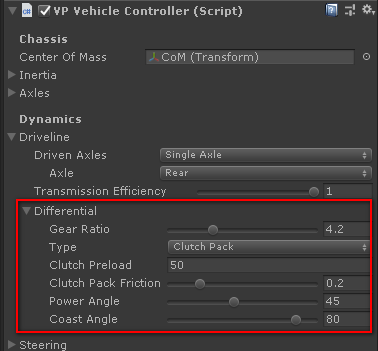Gear Ratio
The input to output reduction ratio of the differential. Car specifications commonly refer to this ratio as Final Ratio. This setting is common to all differential types.

#### Open #

A standard open differential, as mounted in most commercial vehicles.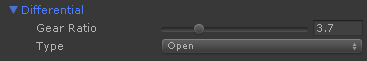Video: How a Differential works?

#### Locked #

A spool forcing both outputs to rotate at the same rate, with all the side effects.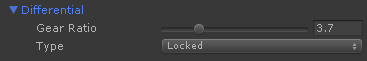Locked differentials are typically used in drifting. In normal situations the car will typically refuse to steer.

#### Viscous #

Outputs are coupled together based on the parameters. The lock state and and the torque transfer depend on the difference of speed between both outputs.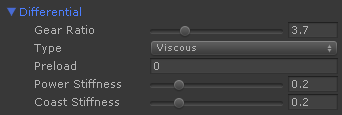The minimum torque that couples both outputs.
Power Stiffness (%)
Stiffness of the coupling between both outputs when the input applies forward torque (power). 0.0 is an open differential. 1.0 is a locked differential.
Coast Stiffness (%)
Stiffness of the coupling between both outputs when the input applies reverse torque (coast). 0.0 is an open differential. 1.0 is a locked differential.

The viscous differential can be set up to mimic an open differential, or in the extreme, a spool, and virtually any combination in between. Examples:

Type Preload Power Stiffnes Coast Stiffness
Open 0 0 0
Locked (any) 1 1
Viscous by lock ratio (%) 0 % < 1 % < 1
Viscous by torque (Nm) torque 0 0

#### Clutch Pack #

Lock state and torque transfer depend on the input torque engaging a clutch pack. They behave as open differential when no torque is applied or one of the outputs has no resistance (i.e. when a wheel is lifted).

Differentials in this category include Salisbury, Limited Slip (1-way, 1.5-way, 2-way), Powerflow, Torque-Lock, VariLock...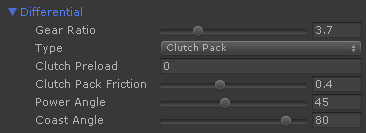Minimum torque transfer provided by the clutch pack. Outputs are coupled by this value.
Clutch Pack Friction
Coefficient of friction of the clutch pack.
Power Angle (degrees)
Ramp angle applied when the input receives forward torque (power).
Coast Angle (degrees)
Ramp angle applied when the input receives reverse torque (coast).

#### Torque Bias #

Input torque is biased among outputs proportionally to the output with less resistance. The ratio defines how much torque can be biased to the output with most resistance. They behave as open differential when no torque is applied or one of the outputs has no resistance.

Differentials in this category include Torsen, Quaife, Truetrac, torque biasing / torque sensing differentials, limited slip by ratio differentials...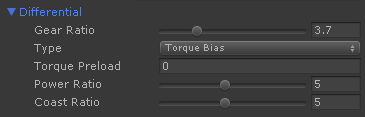Minimum torque that couples both outputs.
Power Ratio (n)
Ratio n:1 of torque that is applied to the output with most resistance when the input receives forward torque (power).
Coast Ratio (n)
Ratio n:1 of torque that is applied to the output with most resistance when the input receives reverse torque (coast).

For example, a 4:1 Torsen differential can be configured by setting 0 preload and both ratios to 4.

## Diagnosing the differential effects #

The best way is using the wheel spin chart of the Performance Display component. It shows the wheel circumference speed for each wheel. You can see the difference of speed between left and right drive wheels, and compare how the differential affects it in the different situations.

Here's the chart for an open differential when heavily accelerating after a corner. The inner wheel losses traction and reduces the acceleration: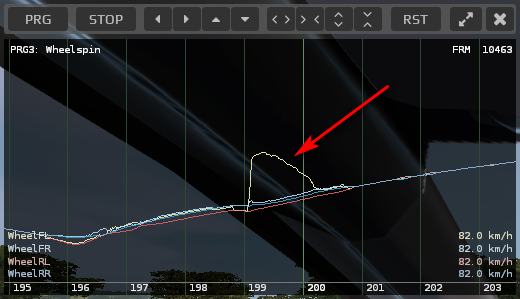The same situation with the default clutch pack differential. After a minimum slip the differential locks and allows both drive wheels to gain traction at the same time, providing better acceleration: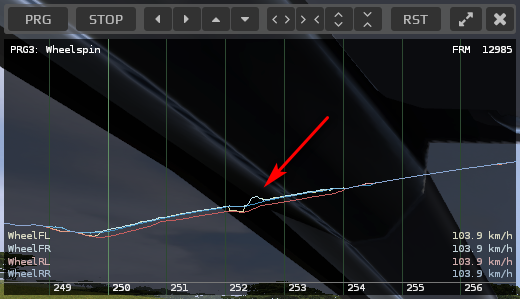# Scripting reference #

### Differential.Settings #

namespace VehiclePhysics
{
public class Differential : Block
{
public enum Type { Open, Locked, Viscous, ClutchPack, TorqueBias };

[Serializable]
public class Settings
{
// Type of differential

public Type type = Type.Viscous;

// Ratio of the differential gear

[Range(1,12)]
public float gearRatio = 3.7f;

// Viscous type settings:
//
// Preload: torque (Nm) required to rotate the gears in the differential
// Stiffness: lock ratio (%) among both outputs
//
//      0.0 = Open differential. No torque transfer among the outputs.
//      1.0 = Locked (spool, live axle). Outputs behave like linked with a rigid rod.

[Range(0,1)]
public float powerStiffness = 0.2f;
[Range(0,1)]
public float coastStiffness = 0.2f;

// Clutch-pack type settings:
//
// Preload: torque (Nm) required to rotate the gears in the differential
// clutchPackFriction: power to friction ratio of the clutch pack (depends on number and compound)
// powerAngle: ramp angle (º) for the power mode (~30-80º)
// coastAngle: ramp angle (º) for the coast mode (~30-80º)
//
// Note: clutch friction values above 0.5 and low ramp angles (<45) could lock the
// differential even with the wheel inertia only (one wheel lifted). Not very realistic.

[Range(0,1)]
public float clutchPackFriction = 0.4f;
[Range(10,90)]
public float powerAngle = 45.0f;
[Range(10,90)]
public float coastAngle = 80.0f;

// Torque bias settings:
//
// Preload: torque (Nm) required to rotate the gears in the differential
// Ratio: proportion of torque that can be transferred from one output to another.
//
//      1 = 1:1 Open differential. Both outputs receive the less resistance torque.
//      2 = 2:1 torque bias. Up to twice the torque of the least resistance output
//          can be transferred to the most resistance output. Torque split 66% - 33%.
//      5 = 5:1 torque bias. Torque split goes up to 20% - 80%
//          This is a Torsen differential (5:1).